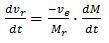# Understanding rocket motion

Hi there
I'm having a bit trouble understanding the rocket motion and how the find the velocity, acceleration and travelheight as a function of time.
First, i assume that the velocity of the ejected mass relative to the rocket is constant. Secondly, i assume that the rocket mass changes with a constant rate. I'm working with the motion in a single dimension.

I have derived the rocket motion equation considering the acceleration of the rocket to be:where vr is the velocity of the rocket, ve is the exhaust velocity, Mr is the mass of the rocket and dM/dt is the change in mass over the interval dt.

So - how do i find:

The velocity as a function of time
The acceleration as a function of time
The travelheight as a function of time